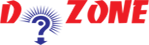﻿ Python Program: How to find a Leap Year?## Python Program To find a Leap Year

Here in this Python tutorial, you will check whether a year is leap year or not. We will use if...else statement to solve this problem.

We all know that if a year is completely divisible by 4 then it is a Leap Year. If a Leap Year is a century year (years ending with 00) then it is a leap year only whne it is completely divisible by 400.

``````
2018 is not a Leap Year
2006 is not a Leap Year
2020 is a Leap Year
2000 is a Leap Year
```
```
``````
Python Code
# Python program to check if the input year is a leap year or not

year = 2000

# To get year (integer input) from the user
# year = int(input("Enter a year: "))

if (year % 4) == 0:
if (year % 100) == 0:
if (year % 400) == 0:
print("{0} is a leap year".format(year))
else:
print("{0} is not a leap year".format(year))
else:
print("{0} is a leap year".format(year))
else:
print("{0} is not a leap year".format(year))

Output
2000 is a leap year
```
```

So, if you want to test the program, then change the value of the year in the python code and run it.

## Other Python Programs

PythonTraining, 258-FF , Katewa Nagar, New Sanganer Rd, Jaipur, 302019